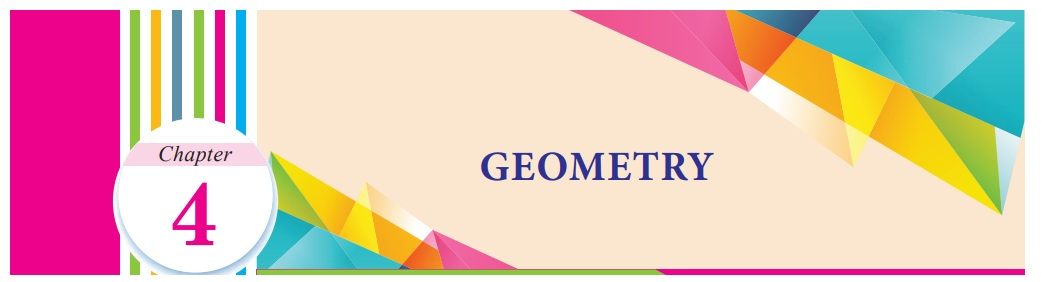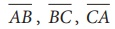Home | | Maths 7th Std | Geometry

# Geometry

Learning Objectives ● To apply angle sum property of triangles. ● To understand the concept of congruency of triangles. ● To know the criteria for congruence of triangles.

Chapter 4

GEOMETRYLearning Objectives

● To apply angle sum property of triangles.

● To understand the concept of congruency of triangles.

● To know the criteria for congruence of triangles.

Recap

Triangles

In first term we studied about different types of angles made by intersecting lines and parallel lines with transversals. Further we learnt about triangles, types of triangles and properties of triangles. In this term we are going to apply the properties of triangles.

A triangle is a closed figure formed by three line segments. It has three vertices, three sides and three angles.In the triangle ABC (Fig 4.1) vertices are A,B,C, the sides areand the angles are CAB , ABC , BCA . We have already learnt to classify triangles based on sides and angles.

The classification of triangles are shown below.In any triangle drawn by joining three non-collinear points, the sum of the lengths of any two sides is greater than the length of the third side. This property is called as Triangle inequality.

To verify this property, let us consider three types of triangles based on angles.For each of these triangles the following statements are true.

1. a + b > c

2. b + c > a

3. c + a > b

This property is also true for three types of triangles based on sides.

Try these

Anwser the following questions.

1. Triangle is formed by joining three non–collinear points.

2. A triangle has three vertices and three sides.

3. A point where two sides of a triangle meet is known as Vertex of a triangle.

4. Each angle of an equilateral triangle is of 60° measure.

5. A triangle has angle measurements of 29°, 65° and 86°. Then it is _______ triangle.

(i) an acute angled (ii) a right angled (iii) an obtuse angled (iv) a scalene

6. A triangle has angle measurements of 30°, 30° and 120°. Then it is _______ triangle.

(i) an acute angled (ii) scalene (iii) obtuse angled (iv) right angled

7. Which of the following can be the sides of a triangle?

(i) 5,9,14 (ii) 7,7,15 (iii) 1, 2, 4 (iv) 3, 6, 8

(i) 5,9,14 5+9 = 14, 14 = 14 can be side of the triangle

(ii) 7, 7,15 7+7 = 14 < 15 can’t be side of the triangle

(iii) 1,2,4 1+ 2 = 3< 4 can’t be side of the triangle can be side of the triangle

(iv) 3, 6,8 3 + 6 = 9 > 8 can be side of the triangle

(iv) 3, 6,8

3 + 6 = 9 > 8 can be side of the triangle

8. Ezhil wants to fence his triangular garden. If two of the sides measure 8 feet and 14 feet then the length of the third side is __________

(i) 11 ft (ii) 6 ft (iii) 5 ft (iv) 22 ft

9. Can we have more than one right angle in a triangle?

No, it cannot.

10. How many obtuse angles are possible in a triangle?

One.

11. In a right triangle, what will be the sum of other two angles?

90°

12. Is it possible to form an isosceles right angled triangle? Explain.Yes. It is possible.

Side AB = Side BC=3cm

B = 90°

Introduction

Triangles are an effective tool for construction and architecture. They are used in the design of buildings and other structures for more strength and stablility. The knowledge of the properties of triangles is essential to understand its use in architecture. The triangle’s use in architecture dates back more years than other common shapes like dome, arch, cylinder. Usage of triangles even predates the wheel. The most sturdy of the triangles are equilateral and isosceles; their symmetry aids in distributing weight.

This chapter is a continuation of properties of triangles that we studied in class VI.

MATHEMATICS ALIVE - Geometry in Real LifeTags : Term 2 Chapter 4 | 7th Maths , 7th Maths : Term 2 Unit 4 : Geometry
Study Material, Lecturing Notes, Assignment, Reference, Wiki description explanation, brief detail
7th Maths : Term 2 Unit 4 : Geometry : Geometry | Term 2 Chapter 4 | 7th Maths